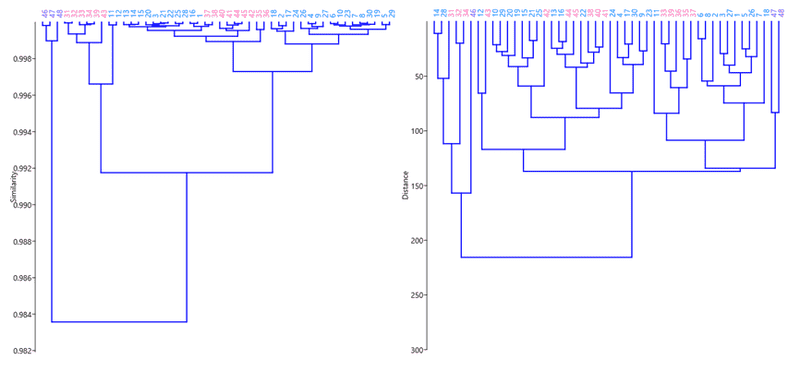# Principal component analysis (PCA) coefficients

• I
FactChecker
Gold Member
I have not followed a lot of this thread, and do not have the background to understand much of it. For my own curiosity, I wonder why you are trying to use PCA for this problem. My general classification of problems and the appropriate statistical analysis leads me to suggest regression analysis:

Analysis Types:
• Looking for descriptions of similarities of a group: Principle Component Analysis (PCA); Factor Analysis
• Looking for ways to define distinguishable groups (without preconcieved grouping): Cluster analysis
• Looking for explanations and predictors for a known difference: Regression analysis; Analysis of Variance (ANOVA)

Hi @FactChecker,

My spectrophotometric measurements are based on 1350 wavelengths/variables. The aim of my project is to differentiate plants based on a much smaller subset of wavelengths. At the present, I want to know what the most significant variables are. When plotting the results of a PCA, if the separation between two known groups is in terms of a given PC, then plotting the loadings of that PC against variables (as in post #23) tells you what variables are most significant when it comes to distinguishing. The variables for which the loadings are largest in absolute value are the variables where the most important distinguishing features occur (at least that is my understanding). Do you believe I am on the right track?

As I understand it, cluster analysis doesn't really give this information. For instance, the following are the dendrograms I made based on Pearson's correlation on the left and Euclidean distance on the right (by the way, do you know why is the former such a better distance metric for classifying the spectra?). The colours indicate the actual group membership of a measurement.Do you think regression analysis does a better job of telling you what the best variables are?

#### Attachments

FactChecker
Gold Member
Hi @FactChecker,

My spectrophotometric measurements are based on 1350 wavelengths/variables. The aim of my project is to differentiate plants based on a much smaller subset of wavelengths. At the present, I want to know what the most significant variables are. When plotting the results of a PCA, if the separation between two known groups is in terms of a given PC, then plotting the loadings of that PC against variables (as in post #23) tells you what variables are most significant when it comes to distinguishing. The variables for which the loadings are largest in absolute value are the variables where the most important distinguishing features occur (at least that is my understanding). Do you believe I am on the right track?

As I understand it, cluster analysis doesn't really give this information. For instance, the following are the dendrograms I made based on Pearson's correlation on the left and Euclidean distance on the right (by the way, do you know why is the former such a better distance metric for classifying the spectra?). The colours indicate the actual group membership of a measurement.

View attachment 237294

Do you think regression analysis does a better job of telling you what the best variables are?
IMHO, yes. Stepwise regression analysis (see https://en.wikipedia.org/wiki/Stepwise_regression) is specifically designed to determine the best variables to distinguish between values of a dependent variable. So yes, it is made exactly for that. Your dependent variable can be a 0,1 variable which indicates the plant type. A forward stepwise regression would start with the single variable (wavelength) that does the most to determine the plant type. Then, having accounted for the first variable, it would look for the second variable which does the most to add accuracy to the determination. It continues like that till there are no other variables which are statistically worth adding. IMHO, the best version is "bidirectional elimination". After several variables have been added to the model, the early variables may not add much that a combination of the later variables do not already do. If the early variable is no longer statistically significant, the bidirectional elimination algorithm will remove it.

I have assumed that you are looking for the variables that distinguish between two plant types, not more. That allows you to define a 0,1 variable indicating the plant type. If there are more than two plant types which you want to analyze in the same model, that is different and my recommendation would have to be re-evaluated.

Last edited:
•roam
Stephen Tashi